+

# Shapes of Distributions

Author: Sophia Tutorial
##### Description:

Describe shapes of distributions.

(more)

Sophia’s self-paced online courses are a great way to save time and money as you earn credits eligible for transfer to many different colleges and universities.*

No credit card required

46 Sophia partners guarantee credit transfer.

299 Institutions have accepted or given pre-approval for credit transfer.

* The American Council on Education's College Credit Recommendation Service (ACE Credit®) has evaluated and recommended college credit for 33 of Sophia’s online courses. Many different colleges and universities consider ACE CREDIT recommendations in determining the applicability to their course and degree programs.

Tutorial
what's covered
This tutorial will cover the different shapes that distributions can take. Our discussion breaks down as follows:

1. Distribution
2. Symmetric Distribution
3. Skewed Distribution
4. Uniform Distribution
5. Unimodal Distribution
6. Bimodal Distribution
7. Multimodal Distribution

## 1. Distribution

A distribution is a way to visually show how many times a variable takes a certain value.

While distribution displays the values the variable takes and how often, shape describes the data points as a whole. This tutorial will use qualifying descriptors to identify how the distribution of a data set can look when graphed.

term to know

Distribution
A display of data that shows the values the data take and how often those values occur.

## 2. Symmetric Distribution

A symmetric distribution will have the same mean as its median. If plotted, it will look like two mirror images on the same plot.

Here are examples of symmetric distribution: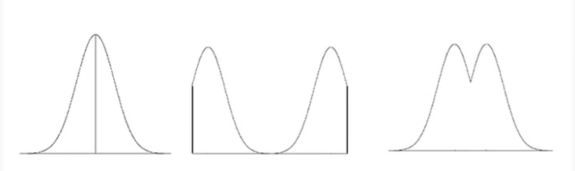In the graph on the far left, for example, the line in the center of the graph is the mirror line, and it represents both the mean and the median of this distribution.

Symmetrical distribution doesn't happen too often. Only a few distributions are actually truly symmetric. Often we get distributions that look something like this: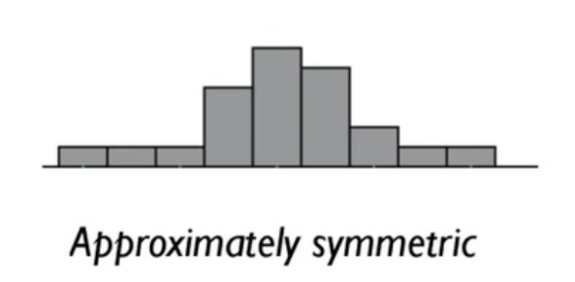Although this distribution is close to being symmetrical, it is not exactly symmetric.

Note that when you say the word symmetric, you must mean exactly. Thus qualifiers like approximately symmetric, roughly symmetric, or nearly symmetric, are necessary to make it clear when a distribution is nearly, but not exactly, symmetric.

term to know

Symmetric Distribution
A distribution where the mean and median are the same. It will appear to have a "mirror line" at the median of the distribution.

## 3. Skewed Distribution

Certain distributions aren't even close to being symmetric. Many asymmetric distributions are called skewed distributions.

These distributions are characterized by a hump, which is sort of a dense grouping with lots of points at certain values and some values that only have a few occurrences. The part of the distribution with fewer occurrences is called a tail. The tail occurs to one side of the median of the distribution. These distributions look like this: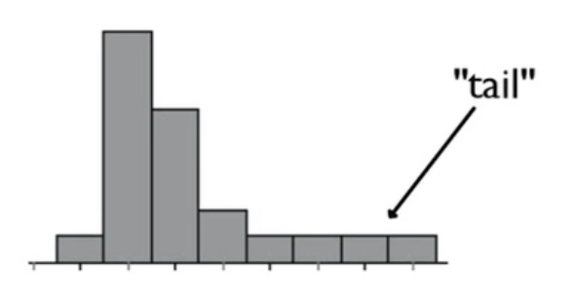There are two ways that a distribution can be skewed.

Skewed Distributions
Right-Skewed
(Positively Skewed)
Tail is on the right side of the median

Right is more positive on the number line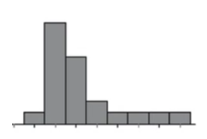Left-Skewed
(Negatively Skewed)
Tail is on the left side of the median

Left is more negative on the number line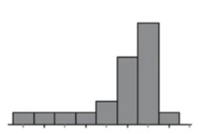terms to know

Skewed Distribution
A distribution where the majority of values are on one side of the distribution, and there are only a few values on the other.
Skewed Right (Positively Skewed) Distributions
A distribution where the majority of values are low, and there only a few high values that form a "tail" to the right of the median.
Skewed Left (Negatively Skewed) Distribution
A distribution where the majority of values are high, and there only a few low values that form a "tail" to the left of the median.

## 4. Uniform Distribution

When all values are equally distributed, then the shape is referred to as being in uniform distribution. Here is an example of uniform distribution: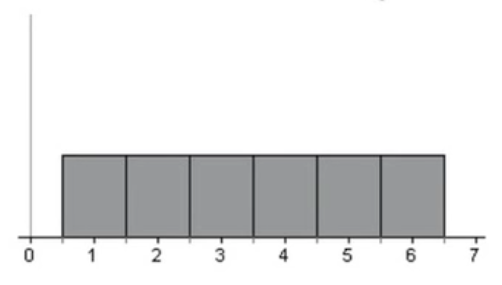Uniform distributions are a certain kind of symmetric distribution. Imagine you put a line of symmetry between the three and four. The two sides would then be symmetric. Moreover, this is a distribution where all the values are equally distributed.

You can also use the same qualifiers for uniform distribution as are used with symmetry.

EXAMPLE

If you rolled a die six times, you might get one 6, one 5, one 4, one 3, one 2, and one 1.

Suppose you rolled the die 600 times, you would expect about 100 of each. However, perhaps you only got 95 1's and 102 2's. The distribution will look almost uniform, so we can use those words like “approximately,” “nearly,” or “almost” uniform in place of the word “exactly” uniform.

term to know

Uniform Distribution
A distribution where all values are equally likely.

## 5. Unimodal Distribution

Often distributions will have a clear peak to their shape. They will peak in just one place on the distribution.

In the table below, each graph has a clear peak, so all of these are called unimodal distributions.

Unimodal Distributions
Peak in the Center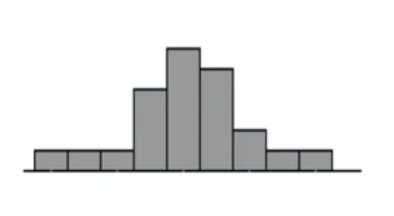Peak to the Right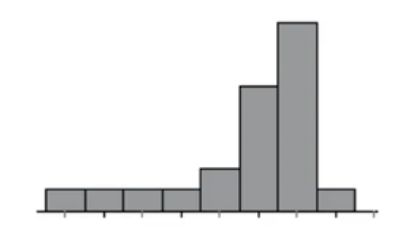Peak to the Left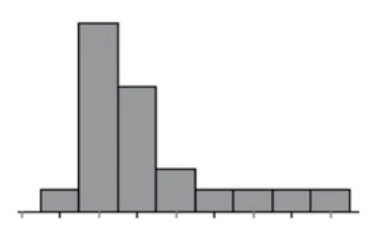hint
The tallest bar is called the mode.

term to know

Unimodal/Single-Peaked Distribution
A distribution where one value or bin contains more data than the other values or bins.

## 6. Bimodal Distribution

You might have a distribution that has two distinct regions with lots of data points and a gap in the middle. When this happens, two peaks form on the distribution. These are both called modes, and a distribution like this is called bimodal distribution.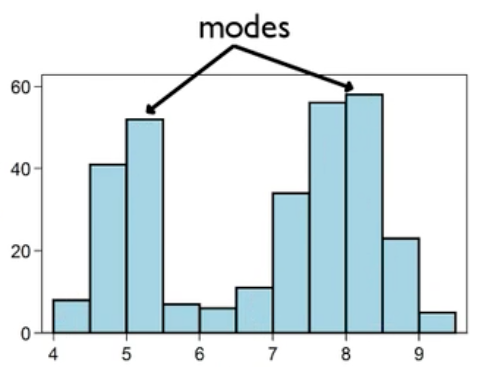Technically, there's only one bin that is the mode: the very tallest bar. However, in the above graph, there are two bins that are the tallest relative to the others around them--also known as local modes.

Now, sometimes you have a distribution that appears bimodal, like the graph below: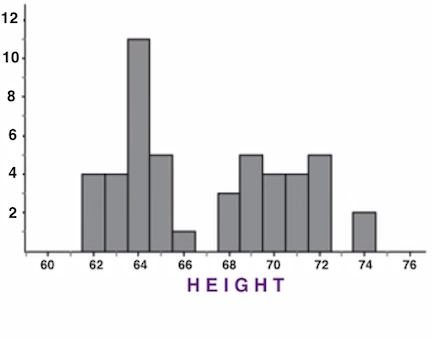Even though it appears to be bimodal, upon further examination of heights, it's possible that you have two different distributions that happen to be graphed on the same set of axes (see below).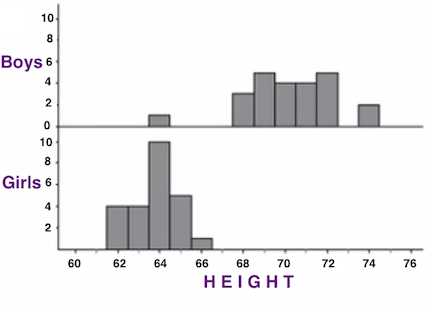There might be some hidden variable that causes the bi-modality. When viewed separately, you end up with two unimodal distributions that just happened to be graphed on the same set of axes.

term to know

Bimodal Distribution
A distribution where there are two distinct values or bins that contain more data than the others, usually separated by a gap.

## 7. Multimodal Distribution

Any distribution with more than two peaks is called a multimodal distribution. This distribution, for instance, has four peaks: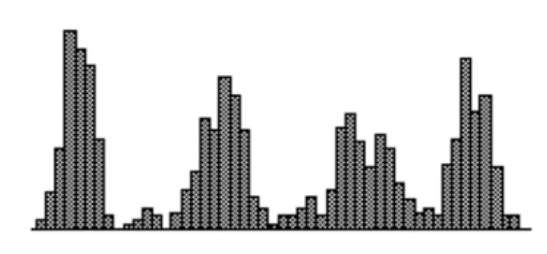You can have the same issue with this type of distribution as you did with the bimodal distribution, in that it may be multiple distributions graphed on the same set of axes.

big idea
Uni means one, bi means two, and modal means the number of modes each distribution has.

term to know

Multimodal Distribution
A distribution where there are many values or bins that contain more data than other nearby bins, usually separated by gaps.

summary
Distributions, when graphed, have many descriptors that we can use to describe their shape. Symmetric distributions visually have mirror halves, and mathematically they have the same mean and median. Uniform distributions are a specific type of a symmetric distribution that are visually very flat. Skewed distributions have a hump on one side of the median and a tail on the other side of the median; if the tail is on the right side of the median, it is called skewed to the right, or positively skewed, and if the tail is to the left of the median, it is skewed to the left, or negatively skewed. Some distributions are unimodal, or single-peaked distributions. Others are bimodal, which means they are clearly double-peaked, and some are multimodal, with more than two peaks. Sometimes, a bimodal distribution is simply two unimodal distributions graphed together.

Good luck!

Source: Adapted from Sophia tutorial by Jonathan Osters.

Terms to Know
Bimodal Distribution

A distribution where there are two distinct values or bins that contain more data than the others, usually separated by a gap.

Distribution

A way to visually display the values a variable takes and how often it takes each value.

Multimodal Distribution

A distribution where there are many values or bins that contain more data than other nearby bins, usually separated by gaps.

Skewed Distribution

A distribution where the majority of values are on one side of the distribution, and there are only a few values on the other.

Skewed Left (Negatively Skewed) Distribution

A distribution where the majority of values are high, and there only a few low values that form a "tail" to the left of the median.

Skewed Right (Positively Skewed) Distributions

A distribution where the majority of values are low, and there only a few high values that form a "tail" to the right of the median.

Symmetric Distribution

A distribution where the mean and median are the same. It will appear to have a "mirror line" at the median of the distribution.

Uniform Distribution

A distribution where all values are equally likely.

Unimodal/Single-Peaked Distribution

A distribution where one value or bin contains more data than the other values or bins.

Rating